# 1 Auction selling price of antique grandfather clocks

The data include the selling price in pounds sterling at auction of 32 antique grandfather clocks, the age of the clock in years, and the number of people who made a bid. In the sections below, describe the relationship between variables and develop a model for predicting selling Price given Age and Bidders.

library(tidyverse)

str(dat_auction)
tibble [32 x 3] (S3: spec_tbl_df/tbl_df/tbl/data.frame)
$Age : num [1:32] 127 115 127 150 156 182 156 132 137 113 ...$ Bidders: num [1:32] 13 12 7 9 6 11 12 10 9 9 ...
\$ Price  : num [1:32] 1235 1080 845 1522 1047 ...
- attr(*, "spec")=
.. cols(
..   Age = col_double(),
..   Bidders = col_double(),
..   Price = col_double()
.. )
summary(dat_auction)
      Age           Bidders           Price
Min.   :108.0   Min.   : 5.000   Min.   : 729
1st Qu.:117.0   1st Qu.: 7.000   1st Qu.:1053
Median :140.0   Median : 9.000   Median :1258
Mean   :144.9   Mean   : 9.531   Mean   :1327
3rd Qu.:168.5   3rd Qu.:11.250   3rd Qu.:1561
Max.   :194.0   Max.   :15.000   Max.   :2131  

## 1.1(1 p) Scatterplot matrix

In a scatterplot matrix below interpret the relationship between each pair of variables. If a transformation is suggested by the plot (that is, because there is a curved relationship), also plot the data on the transformed scale and perform the following analysis on the transformed scale. Otherwise indicate that no transformation is necessary.

library(ggplot2)
library(GGally)
p <- ggpairs(dat_auction)
print(p)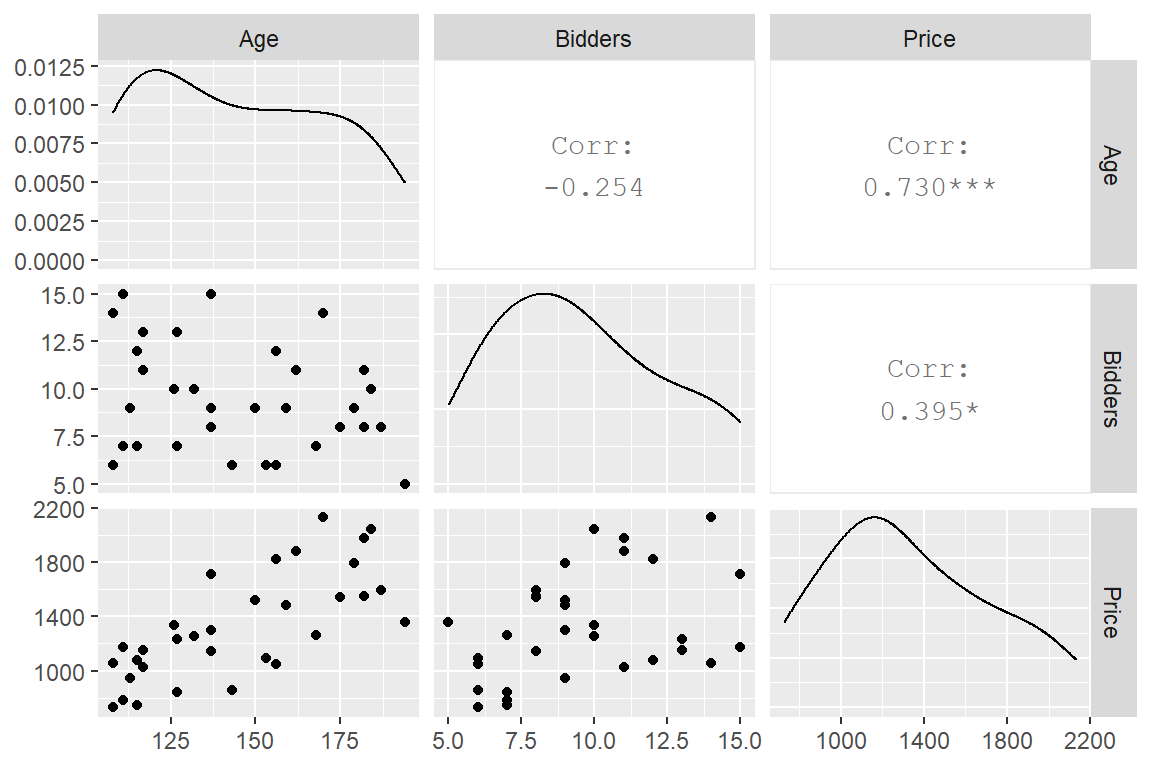## 1.2(1 p) Correlation matrix

Below is the correlation matrix and tests for the hypothesis that each correlation is equal to zero. Interpret the hypothesis tests and relate this to the plot that you produced above.

# correlation matrix and associated p-values testing "H0: rho == 0"
#library(Hmisc)
Hmisc::rcorr(as.matrix(dat_auction))
          Age Bidders Price
Age      1.00   -0.25  0.73
Bidders -0.25    1.00  0.39
Price    0.73    0.39  1.00

n= 32

P
Age    Bidders Price
Age            0.1611  0.0000
Bidders 0.1611         0.0254
Price   0.0000 0.0254        

## 1.3(1 p) Plot interpretation

Below are two plots. The first has $$y =$$ Price, $$x =$$ Age, and colour = Bidders, and the second has $$y =$$ Price, $$x =$$ Bidders, and colour = Age. Interpret the relationships between all three variables, simultaneously. For example, say how Price relates to Age, then also how Price relates to Bidders conditional on Age being a specific value.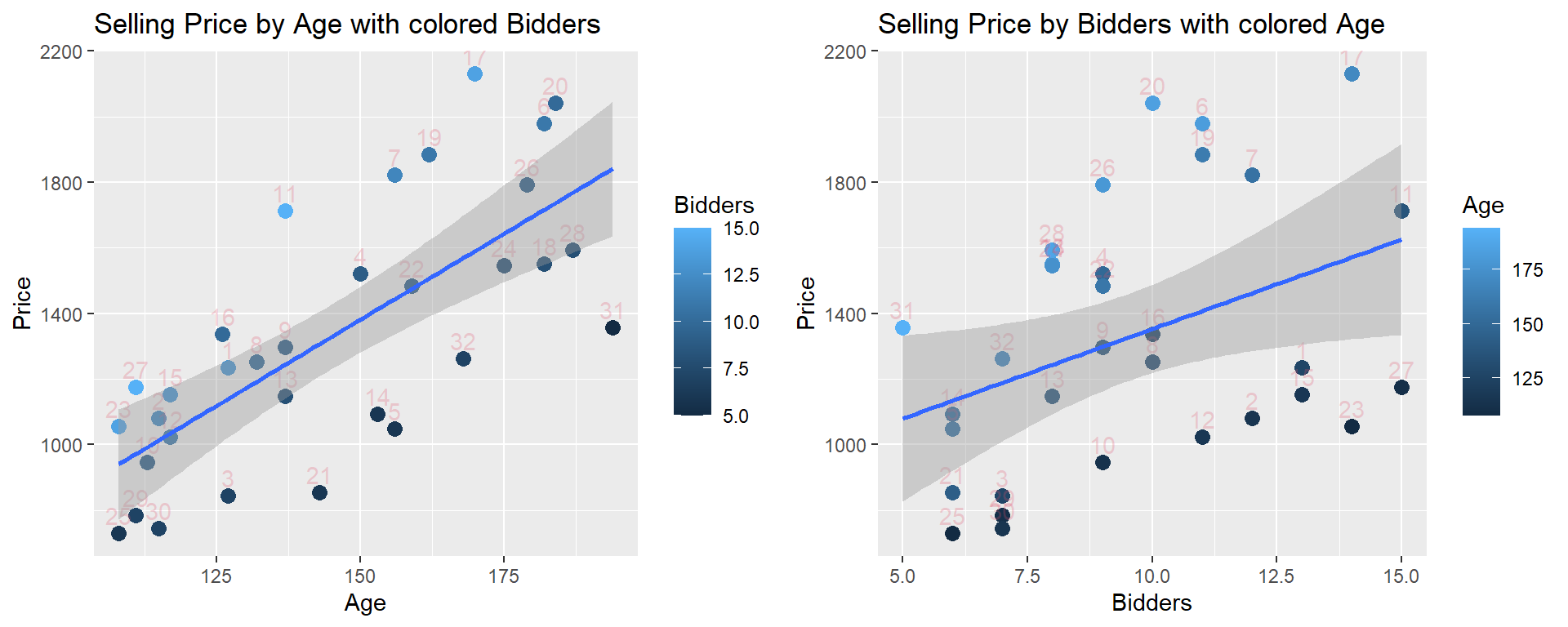## 1.4(2 p) Multiple regression assumptions (assessing model fit)

Below the multiple regression is fit. Start by assessing the model assumptions by interpretting what you learn from the first six plots (save the added variable plots for the next question). If assumptions are not met, attempt to address by transforming a variable and restart at the beginning using the new transformed variable.

# fit the simple linear regression model
lm_p_a_b <- lm(Price ~ Age + Bidders, data = dat_auction)

Plot diagnostics.

# plot diagnostics
lm_diag_plots(lm_p_a_b, sw_plot_set = "simpleAV")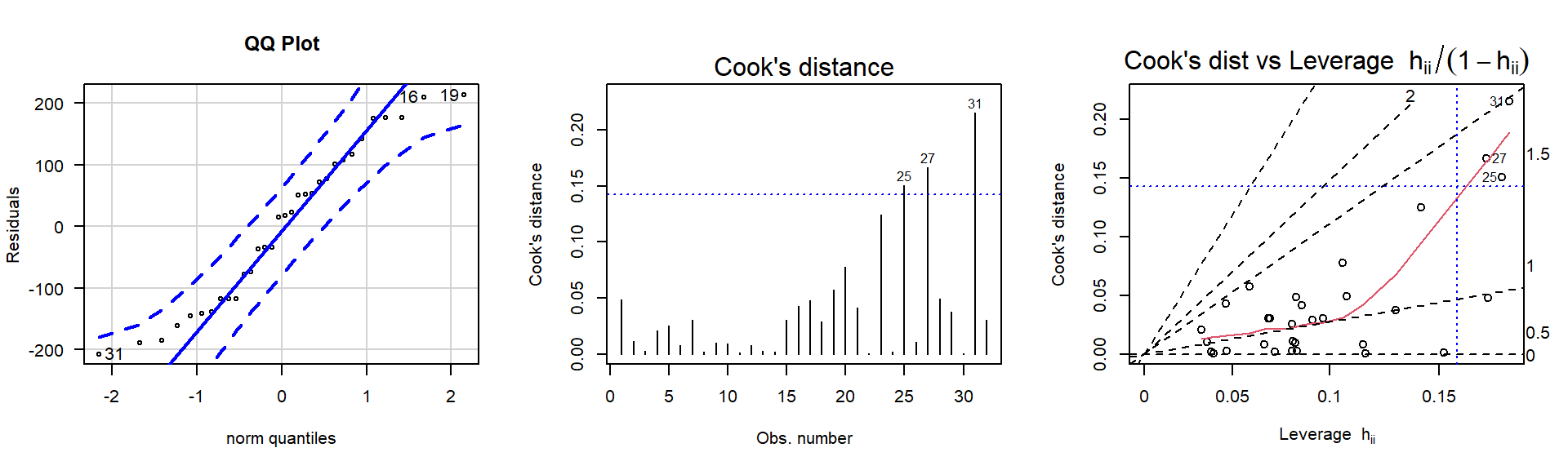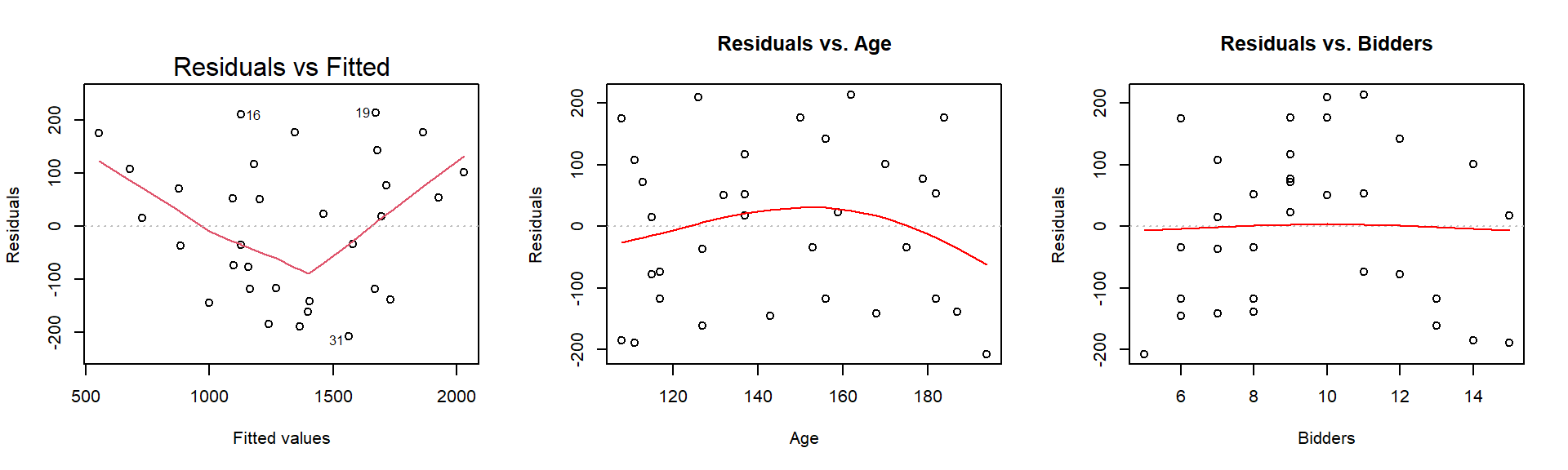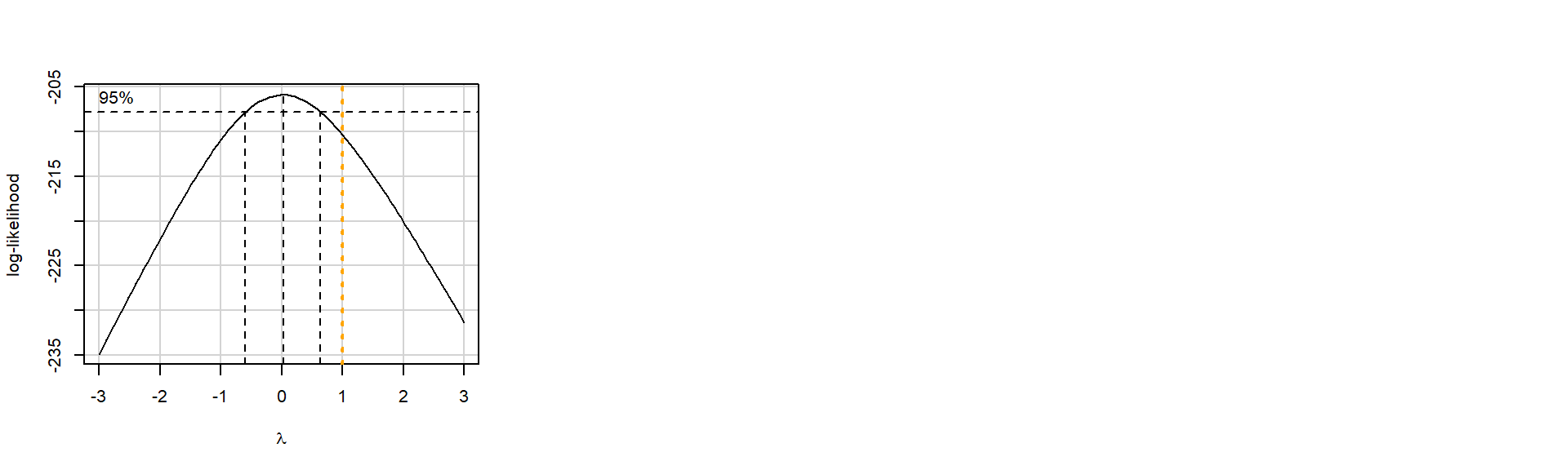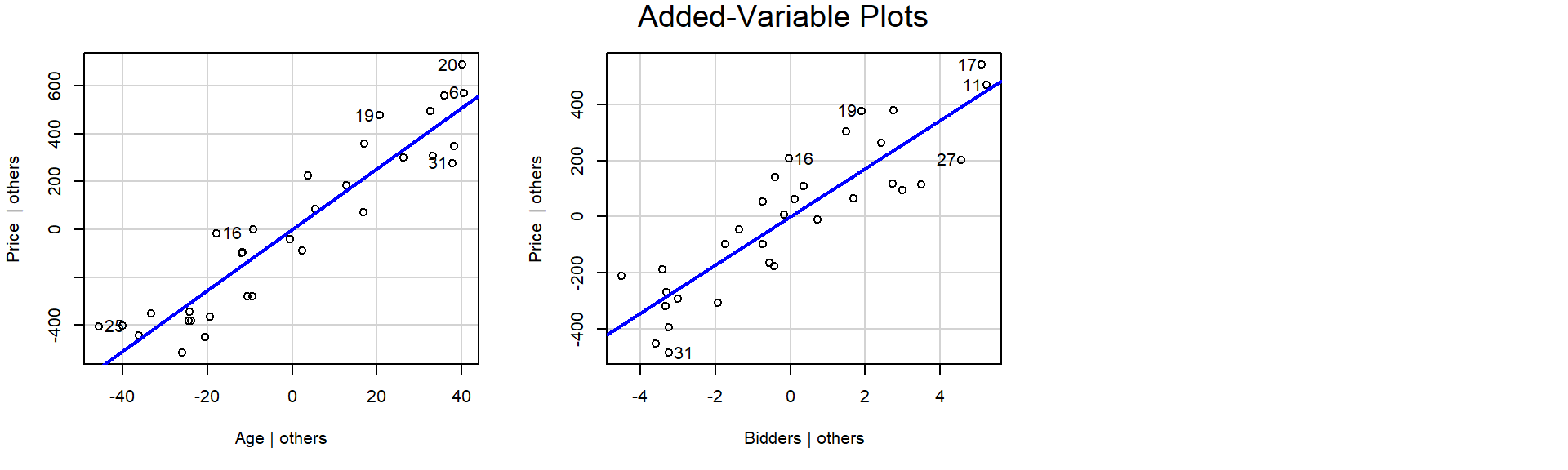### 1.4.1 Solution

From the diagnostic plots above,

## 1.5(1 p) Added variable plots

Use partial regression residual plots (added variable plots) to check for the need for transformations. If linearity is not supported, address and restart at the beginning.

## 1.6(1 p) Multiple regression hypothesis tests

State the hypothesis test and conclusion for each regression coefficient.

# fit the simple linear regression model
lm_p_a_b <- lm(Price ~ Age + Bidders, data = dat_auction)
# use summary() to get t-tests of parameters (slope, intercept)
summary(lm_p_a_b)

Call:
lm(formula = Price ~ Age + Bidders, data = dat_auction)

Residuals:
Min     1Q Median     3Q    Max
-207.2 -117.8   16.5  102.7  213.5

Coefficients:
Estimate Std. Error t value Pr(>|t|)
(Intercept) -1336.7221   173.3561  -7.711 1.67e-08 ***
Age            12.7362     0.9024  14.114 1.60e-14 ***
Bidders        85.8151     8.7058   9.857 9.14e-11 ***
---
Signif. codes:  0 '***' 0.001 '**' 0.01 '*' 0.05 '.' 0.1 ' ' 1

Residual standard error: 133.1 on 29 degrees of freedom
Multiple R-squared:  0.8927,    Adjusted R-squared:  0.8853
F-statistic: 120.7 on 2 and 29 DF,  p-value: 8.769e-15

## 1.7(1 p) Multiple regression interpret coefficients

Interpret the coefficients of the multiple regression model.

## 1.8(1 p) Multiple regression $$R^2$$

Interpret the Multiple R-squared value.

## 1.9(1 p) Summary

Summarize your findings in one sentence.

### 1.9.1 Solution

## Aside: I generally recommend against 3D plots for a variety of reasons.
## However, here's a 3D version of the plot so you can visualize the surface fit in 3D.
## I will point out a feature in this plot that we wouldn't see in other plots
## and it would typically only be detected by careful consideration
## of a "more complicated" second-order model that includes curvature.

# library(rgl)
# library(car)
# scatter3d(Price ~ Age + Bidders, data = dat_auction)